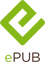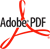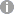# Unified Non-Local Relativistic Theory of Transport Processes

Elsevier (Verlag)
• 1. Auflage
• |
• erschienen am 21. August 2016
• |
• 466 Seiten

 E-Book | ePUB mit Adobe DRM | Systemvoraussetzungen E-Book | PDF mit Adobe DRM | Systemvoraussetzungen
978-0-444-63858-8 (ISBN)

Unified Non-Local Relativistic Theory of Transport Processes highlights the most significant features of non-local relativistic theory, which is a highly effective tool for solving many physical problems in areas where the classical local theory runs into difficulties. The book provides the fundamental science behind new non-local physics - generalized for relativistic cases and applied in a range of scales - from transport phenomena in massless physical systems to unified theory of dissipative structures.

The book complements the author's previous monograph on Unified Non-Local Theory of Transport Processes (Elsevier, 2015), which is mainly devoted to non-relativistic non-local physics. Nevertheless, the theory as handled in this new work is outlined independently so the book can be studied on its own.

• Comprehensive collection of non-local relativistic theory with examples that could previously only be found scattered in the literature
• Provides applications in quantum non-local relativistic hydrodynamics, quantum solitons in solid matter, and plasmas
• Uses generalized non-local kinetic theory as a highly effective tool for solving many physical problems beyond classical physics
• Presents non-local relativistic physics in many related problems of hydrodynamics, gravity, nonlinear optics, time quantization, and applied mathematics
• Includes concrete mathematical problems that are physically consistent and can be solved and studied both analytically and numerically

Professor Boris V. Alexeev is Head of the Centre of the Theoretical Foundations of Nanotechnology and Head of the Physics Department at the Moscow Lomonosov University of Fine Chemical Technologies, Moscow, Russia. In the 1990s he was Visiting Professor at the University of Alabama, Huntsville, AL, USA, and Visiting Professor at the University of Provence, Marseille, France. Professor Alexeev has published over 290 articles in international scientific journals and 22 books. He has received several honors and awards, and is member of six societies.
 Reihe: Sprache: Englisch Verlagsort: Oxford | Niederlande Verlagsgruppe: Elsevier Science Dateigröße: 16,24 MB Schlagworte: ISBN-13: 978-0-444-63858-8 (9780444638588) ISBN-10: 0-444-63858-X (044463858X)weitere Ausgaben werden ermittelt
• Front Cover
• Unified Non-Local Relativistic Theory of Transport Processes
• Contents
• Preface
• Introduction: Review of the Main Principles of the Unified Nonlocal Theory of Transport Processes
• I.1. The Principles of Nonlocal Physics
• I.2. On the Solution of Fundamental Problems of Nonlocal Statistical Theory of Dissipative Processes
• I.3. Fundamental Postulates of the Special Theory of Relativity
• References
• Chapter 1: Hydrodynamic Form of the Dirac Quantum Relativistic Equation
• 1.1. "Derivation" of the Dirac Equation
• 1.2. Hydrodynamic Form of Dirac Equation in the Absence of an External Field
• 1.3. Hydrodynamic Form of the Dirac Equation in an External Electromagnetic Field
• References
• Chapter 2: Generalized Relativistic Nonlocal Kinetic Equation Without Taking Into Account the External Forces
• 2.1. The Collision Integral
• 2.2. Nonlocal Relativistic Kinetic Equation
• 2.3. Model Generalized Relativistic Kinetic Equation
• References
• Chapter 3: Classical Relativistic Equilibrium Distribution Function and Computation of Equilibrium Values of Some Macroscop ...
• 3.1. The Derivation of the Formula for the Classical Equilibrium Distribution Function
• 3.2. The Calculation of the Number Density
• 3.3. The Calculation of the Energy Density and Pressure
• 3.4. The Calculation of the Density of Entropy
• References
• Chapter 4: Generalized Relativistic Hydrodynamic Enskog Equations Without Regard to External Forces
• 4.1. Derivation of the Continuity Equation
• 4.2. Derivation of the Motion Equation
• 4.3. Derivation of the Energy Equation
• 4.4. Generalized Enskog System of Relativistic Hydrodynamic Equations
• References
• Chapter 5: Generalized Relativistic Hydrodynamic Euler Equations Without Taking Into Account the External Forces
• 5.1. Components of Tensor Physical Quantities in the Local Rest System
• 5.2. The Lorentz Transformation Law for Tensor Physical Quantities in One-Dimensional Case
• 5.3. Generalized Relativistic Euler Equations for One-Dimensional Motion
• 5.4. Generalized One-Dimensional Nonrelativistic Euler Equations as the Asymptotic Behavior of the Relativistic Equations
• 5.5. Generalized Relativistic Euler Equations for Arbitrary Motion
• 5.6. Generalized Nonrelativistic Euler Equations for an Arbitrary Motion as an Asymptotic Form of the Relativistic Equations
• References
• Chapter 6: The Solution of Some Physical Problems on the Basis of the Nonlocal Relativistic Equations Without Taking Into ...
• 6.1. The Propagation of Plane Harmonic Waves of Small Amplitude in the Ultra-Relativistic Medium
• 6.2. Shock Waves in Relativistic Gas
• 6.3. The Collision of Nucleons
• References
• Chapter 7: Nonlocal Relativistic Kinetic Equation and Hydrodynamic Enskog Equations Taking Into Account the External Forces
• 7.1. Nonlocal Relativistic Kinetic Equation Taking Into Account the External Forces
• 7.2. The Derivation of the Continuity Equation
• 7.3. The Derivation of the Motion Equation
• 7.4. The Derivation of the Energy Equation
• 7.5. Generalized System of Relativistic Hydrodynamic Enskog Equations
• 7.6. Generalized System of Relativistic Hydrodynamic Enskog Equations for Multicomponent Mixture
• References
• Chapter 8: Generalized Relativistic Euler Equations Taking Into Account External Forces
• 8.1. Components of Tensor Physical Quantities in the Local Rest System
• 8.2. The Lorentz Transformation Law for Tensor Physical Quantities in One-Dimensional Case
• 8.3. Generalized Relativistic Euler Equations for One-Dimensional Motion
• 8.4. Generalized One-Dimensional Nonrelativistic Euler Equations as the Asymptotic Behavior of the Relativistic Equations
• References
• Chapter 9: Application of the Generalized Relativistic Kinetic and Hydrodynamic Equations to the Study of Graphene
• 9.1. Features of the Band Structure of Graphene
• 9.2. Generalized Kinetic and Hydrodynamic Equations for Graphene in an Electric Field
• 9.3. Wave Transfer in Graphene
• 9.4. The Hall Effect in the Relativistic Nonlocal Theory of Massless Particles
• References
• Chapter 10: Application of Relativistic Nonlocal Physics in the Theory of Gravitational Field Interaction With a Laser Beam
• 10.1. Nonlocal Physics and Gravitational Waves
• 10.2. Basic Equations of Relativistic Nonlocal Physics Having Regard to External Forces
• 10.3. Calculation of the Tensor Moments for the Laser Beam Evolution
• 10.4. Nonlocal Relativistic Equations in the Explicit Form
• 10.5. Wave Solutions for GFR-Interaction
• References
• Chapter 11: Application of the Generalized Relativistic Hydrodynamic Equations to the Study of the Interaction of Planck R ...
• 11.1. About the Influence of Gravitational Field on Radiation
• 11.2. Nonlocal Relativistic Hydrodynamic Equations for Equilibrium Radiation
• 11.3. Local Equilibrium Radiation in Gravitational Field for the One-Dimensional Stationary Case
• 11.4. Self-Similar Solutions of Hydrodynamic Equations for Planck Radiation in Gravitational Field
• References
• Chapter 12: Connection Between Time Quantization in the Frame of Nonlocal Relativistic Physics and Shannon-Nyquist-Kotelnik ...
• 12.1. About the Time Quantization
• 12.2. Systems of Generalized Relativistic Hydrodynamic Equations in the Shannon-Nyquist-Kotelnikov Theory
• 12.3. Shannon-Nyquist-Kotelnikov Theorem as a Consequence of Nonlocal Physics: Time Quantization
• References
• Appendices
• Appendix 1. Elements of the Theory of Tensors
• Appendix 2. Conservation of Phase Volume dx1dx,2dx,3dt Under the Lorentz Transformations
• Appendix 3. The Relativistic Invariance of the Substantial Derivative in One-Dimensional Motion
• Appendix 4. Integration of Four-Dimensional Divergence in Momentum Space
• Appendix 5. Calculation of the TKpK,0 Tensor
• Index
• Back Cover
Schweitzer Klassifikation
Newbooks Subjects
• Naturwissenschaften
•Astronomie
•Astrophysik
• Naturwissenschaften
•Astronomie
•Kosmologie, Urknalltheorie
• Naturwissenschaften
•Physik
•Angewandte Physik
•Astrophysik
DNB DDC Sachgruppen
Dewey Decimal Classfication (DDC)
• Science
•Physics
•Physics
BIC Classifikation
BISAC Classifikation
• Science
•Physics / General
•Mathematical & Computational
• Science
•Physics / General
•Quantum Theory
Warengruppensystematik 2.0Dateiformat: EPUB
Kopierschutz: Adobe-DRM (Digital Rights Management)

Systemvoraussetzungen:

Computer (Windows; MacOS X; Linux): Installieren Sie bereits vor dem Download die kostenlose Software Adobe Digital Editions (siehe E-Book Hilfe).

Tablet/Smartphone (Android; iOS): Installieren Sie bereits vor dem Download die kostenlose App Adobe Digital Editions (siehe E-Book Hilfe).

E-Book-Reader: Bookeen, Kobo, Pocketbook, Sony, Tolino u.v.a.m. (nicht Kindle)

Das Dateiformat EPUB ist sehr gut für Romane und Sachbücher geeignet - also für "fließenden" Text ohne komplexes Layout. Bei E-Readern oder Smartphones passt sich der Zeilen- und Seitenumbruch automatisch den kleinen Displays an. Mit Adobe-DRM wird hier ein "harter" Kopierschutz verwendet. Wenn die notwendigen Voraussetzungen nicht vorliegen, können Sie das E-Book leider nicht öffnen. Daher müssen Sie bereits vor dem Download Ihre Lese-Hardware vorbereiten.

Weitere Informationen finden Sie in unserer E-Book Hilfe.Dateiformat: PDF
Kopierschutz: Adobe-DRM (Digital Rights Management)

Systemvoraussetzungen:

Computer (Windows; MacOS X; Linux): Installieren Sie bereits vor dem Download die kostenlose Software Adobe Digital Editions (siehe E-Book Hilfe).

Tablet/Smartphone (Android; iOS): Installieren Sie bereits vor dem Download die kostenlose App Adobe Digital Editions (siehe E-Book Hilfe).

E-Book-Reader: Bookeen, Kobo, Pocketbook, Sony, Tolino u.v.a.m. (nicht Kindle)

Das Dateiformat PDF zeigt auf jeder Hardware eine Buchseite stets identisch an. Daher ist eine PDF auch für ein komplexes Layout geeignet, wie es bei Lehr- und Fachbüchern verwendet wird (Bilder, Tabellen, Spalten, Fußnoten). Bei kleinen Displays von E-Readern oder Smartphones sind PDF leider eher nervig, weil zu viel Scrollen notwendig ist. Mit Adobe-DRM wird hier ein "harter" Kopierschutz verwendet. Wenn die notwendigen Voraussetzungen nicht vorliegen, können Sie das E-Book leider nicht öffnen. Daher müssen Sie bereits vor dem Download Ihre Lese-Hardware vorbereiten.

Weitere Informationen finden Sie in unserer E-Book Hilfe.